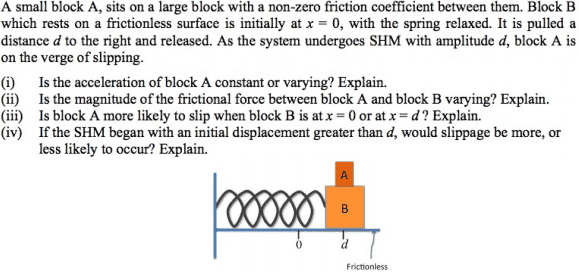# Magnitude of Frictional Force in Simple Harmonic Motion

## Homework StatementI do not fully grasp the concept behind all of these sub questions (i)-(iv).

## Homework Equations

v=wAcos(wt) (SMH)?
Friction Force = Coefficient of Friction * Normal Force

## The Attempt at a Solution

(i) Varying as simple harmonic motion sees varying acceleration as it oscillates forwards, until a stationary point and then backwards.

(ii) Same (not varying) as block A moves with block B, in identical harmonic motion?

(iii) I think this has to do with the result of (ii), which would indicate (ii)'s correct answer is 'varying' but I am not sure why (conceptually).

(iv) as (iii)

## Answers and Replies

jbriggs444
Science Advisor
Homework Helper
F=ma. If a is varying, what can be said about F?

•Rotnort
F=ma. If a is varying, what can be said about F?
I see, very simple. Thank you!

So for (iii), block A would be more likely to slip when block B is at x=d because at x=d block B would have greater acceleration (would it?)

Last edited:
jbriggs444
Science Advisor
Homework Helper
I see, very simple. Thank you!

So for (iii), block A would be more likely to slip when block B is at x=d because at x=d block B would have greater acceleration (would it?)
Yes!